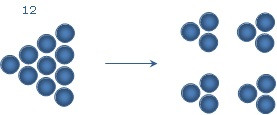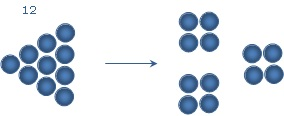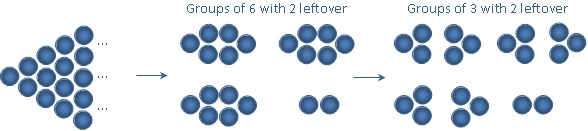# Quarter Wit, Quarter Wisdom: Divisibility UnraveledToday, I will start the topic of Divisibility. We will discuss what divisibility is at a very basic level this week and then move on to remainders in the coming weeks.

So the first question is –- what is division? Don’t tell me what to do to divide a number by another, tell me why you do it. What is it that you are achieving by dividing one number by another? Let me tell you what I think –- I like to think that division is grouping. Not happy? Let’s look at an example then.

When I divide 6 by 2, I am actually just making groups of 2 from 6 and subtracting them.

6 – 2 = 4 (One group of 2 subtracted.)

4 – 2 = 2 (Another group of 2 subtracted.)

2 – 2 = 0 (Third group of 2 subtracted.)

I subtracted three groups so I get 3 as my quotient. Nothing is leftover so remainder is 0.

If I were to divide 14 by 3, I would subtract 4 groups of 3 from 14 giving me a quotient of 4, and 2 will be leftover. Hence, remainder will be 2. Hopefully, this made sense to you. Now let’s look at division from the ‘divisibility angle’.

Is 82 divisible by 4?

What I mean to ask here is that can I subtract groups of 4 from 82 such that nothing is leftover? When I subtract 20 groups of 4 (=80) from 82, I am left with 2. I cannot make any more groups of 4. Since we have a remainder of 2, 82 is not divisible by 4.

I have a fascination for diagrams. It stems in part from the fact that I wanted to be an artist but found that I have no talent in the field, and in part from the fact that I believe that diagrams help people understand the concepts better. On that note, let me draw a few diagrams to help you understand divisibility. Thereafter, you will be able to solve the tricky remainder questions quickly.

Imagine numbers as balls. When I say 7, imagine 7 balls in a group. Let’s start easy: Is 7 divisible by 3? Before answering, think of the following figure:To divide by 3, I have to split 7 into groups of 3. I can make two groups of 3 and then 1 ball is leftover. Hence, when I divide 7 by 3, quotient is 2 and remainder, the ball that could not be put in a group, is 1.

Similarly, what happens when I divide 12 by 3?I get 4 groups and nothing is leftover. (Is this then the diagrammatic representation of 12 = 3*4? Sure.) Then when I divide 12 by 4, I should be able to get 3 groups with nothing leftover.I guess you get the picture!

Now, a question: I have a number that when divided by 6, leaves a remainder of 2. What will be the remainder when the number is divided by 3?

(Do you think I moved on too quickly to too difficult a concept? Not really, just follow me)
So here, I do not know what the number is, but I know that when I make groups of 6 balls each, 2 balls are leftover (as shown in the diagram below). Logically, it follows that when I split each group of 6 balls into two groups of 3 balls, I will still have the same 2 balls remaining.Since there are 2 balls leftover when groups of 3 are made, we can say that when we divide this number by 3, the remainder will still be 2! You don’t have to make the diagram in the exam of course. Just imagine it.

A slight twist on the question above: I have a number that when divided by 6, leaves a remainder of 4. What will be the remainder when the number is divided by 3? Imagine the picture. Just like above but with groups of 6, there are 4 balls leftover. You divide the groups of 6 into groups of 3 – all is well till now – but then, you make another group of 3 from the 4 balls that are leftover. Therefore, only one ball will be leftover. Hence, when you divide the given number by 3, the remainder will be 1!

Try a few more examples and let this theory sink in. We will delve into more details in the coming few weeks.

Karishma, a Computer Engineer with a keen interest in alternative Mathematical approaches, has mentored students in the continents of Asia, Europe and North America. She teaches the GMAT for Veritas Prep in Detroit, Michigan, and regularly participates in content development projects such as this blog!